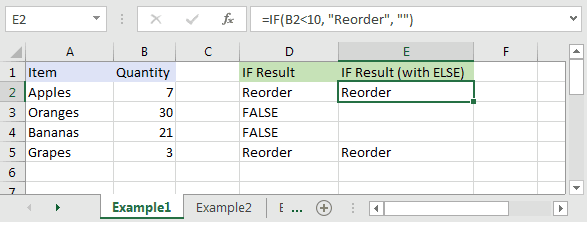## Excel Office

Excel How Tos, Tutorials, Tips & Tricks, Shortcuts

# IF function: Description, Usage, Syntax, Examples and Explanation

## What is IF function in Excel?

IF function is one of logical functions in Microsoft Office Excel that returns one value if the condition is TRUE, or another value if the condition is FALSE.

## Syntax of IF function

The syntax for the IF function in Microsoft Excel is:

`IF( condition, value_if_true, [value_if_false] )`

## IF formula explanation

condition
The value that you want to test.
value_if_true
It is the value that is returned if condition evaluates to TRUE.
value_if_false
Optional. It is the value that is returned if condition evaluates to FALSE.
Also See:   If cell is not blank in Excel

## Returns

The IF function returns value_if_true when the condition is TRUE.
The IF function returns value_if_false when the condition is FALSE.
The IF function returns FALSE if the value_if_false parameter is omitted and the condition is FALSE.

## Example of  IF function

Let’s explore how to use the IF function as a worksheet function in Microsoft Excel.

Also See:   How to get last row in mixed data with blanks in ExcelBased on the Excel spreadsheet above, the following IF examples would return:

```=IF(B2<10, "Reorder", "")
Result: "Reorder"

=IF(A2="Apples", "Equal", "Not Equal")
Result: "Equal"

=IF(B3>=20, 12, 0)
Result: 12```